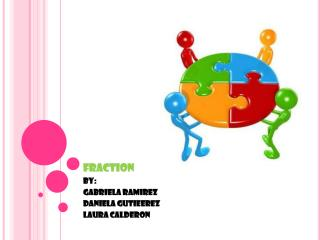DownloadDownload Presentationfraction

# fraction

Télécharger la présentation## fraction

- - - - - - - - - - - - - - - - - - - - - - - - - - - E N D - - - - - - - - - - - - - - - - - - - - - - - - - - -
##### Presentation Transcript

1. fraction By: Gabriela ramirez Daniela gutieerez Laura calderon

2. What is fraction • the fraction is a number, which is obtained of dividing a totality in equal parts. • A fraction is represented mathematically by numbers that they are written one on other one, and they are separate by a straight horizontal line called fractional stripe. fraction al stripe

3. What is fraction • A fraction is the ratio of two whole numbers, or to put it simply, one whole number divided by another whole number. We write a fraction like this:

4. What are the parts that a fraction have For example, break the hexagon into 6 equal pieces Now, we take just 1 piece of the hexagon this is 1 of gright The numerator is the pice that is diferent of a whole says how many equal parts in the whole object

5. how we use fraction in real live • We use fraction in real life like for example when we want to divide a pizza in family because we have to know how many parts we take of the pizza and the same with a cake

6. Improper fractions and mixed numbers • Improper fractions: An Improper fraction has a top number larger than (or equal to) the bottom number Ex: • Mixed Fractions: A whole number and proper fraction together Ex:

7. Equivalent fractions Equivalent fractions are fractions that have the same value or represent the same part of an object. Fractions are determined to be equivalent by multiplying the numerator and denominator of one fraction by the same number. This number should be such that the numerators will be equal after the multiplication

8. Add fractions There are 3 steps for adding fractions. step 1: find the common number of the denominator(l.c.d) step2: multiply the fractions to get the l.c.d step:3 add the results of the step 2.

9. Problem solving 1/2 of the class has blond hair. 1/4 of the class has black hair. 1/4 of the class has brown hair. If there are 32 kids in the class, how many kids have each color hair? 8 16 8 32 32 32 B L O N D h A I r B l A C K H A I r B R O W N H A I r R: R: R: 16 kids 8 kids 8 kids

10. Problem solving Lucy has the ¾ of the age of Tommy and he has 20 years .How many years has lucy? Answear: Lucy ´s age : 20 x ( 3 / 4 ) = 60 / 4 = 15 years

11. Problem solving Georgetown's zoo has two elephants. The male elephant weighs 5/8 of a ton and the female elephant weighs 1/2 of a ton. How much more does the male weigh than the female? step 1: 8 16 24 2 4 6 8 5 step 2: 5 8 1 1 4 1 *4 8 2 2 8 1 8 R: *4 8 more

12. Problem solving This afternoon Wendy left school, rode the bus 1/2 of a mile, and then walked 1/4 of a mile to get home. How many walk Wendy? 1 1 4 8 12 step 1: 4 2 2 4 6 8 1 step 2: 4 1 2 3 *2 2 4 3 4 R: *2 4 MILE

13. conclusion • We learn that there are many kings of fractions and some are easy an some difficult • The fraction can be used in the real life for all an is so utile • Also we learn that we need fractions in our live

14. conclusions • We learn that the line that divide the denominator and numerator is called fractional stripe • We learn to do addition fraction and the steps to do it we learn a lot of fraction

15. conclusion • There are a lot of information that we didn't know • We learn the equivalent fractions mixed numbers and improper fractions !! Thank you for your attention!!